Next: Oscillation of an Elastic Up: Multi-Dimensional Waves Previous: Cylindrical Waves

# Spherical Waves

Consider a spherically symmetric wavefunction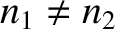, where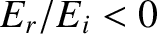is a standard spherical coordinate (Fitzpatrick 2008). Assuming that this function satisfies the three-dimensional wave equation, (537), which can be rewritten (see Exercise 3)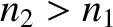(540)

it can be shown (see Exercise 3) that a sinusoidal spherical wave of phase angle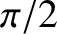, wavenumber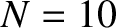, and angular frequency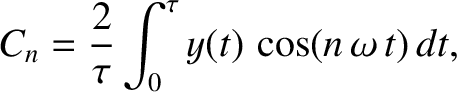, has the wavefunction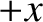(541)

Here,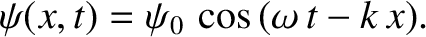is the amplitude of the wave. The associated wavefronts are a set of concentric spheres that propagate radially outward, from their common center (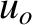), at the phase velocity. The wave amplitude attenuates as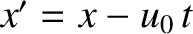. Such behavior can again be understood as a consequence of energy conservation. (The area of a constant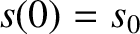surface scales as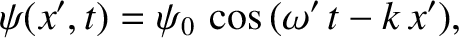, and the power flowing such a surface is proportional to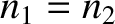.) The spherical wave specified in expression (541) is such as would be generated by a point source located at.Next: Oscillation of an Elastic Up: Multi-Dimensional Waves Previous: Cylindrical Waves
Richard Fitzpatrick 2013-04-08